# conjugatebvarm

Bayesian vector autoregression (VAR) model with conjugate prior for data likelihood

Since R2020a

## Description

The Bayesian VAR model object conjugatebvarm specifies the joint prior or posterior distribution of the array of model coefficients Λ and the innovations covariance matrix Σ of an m-D VAR(p) model. The joint prior distribution (Λ,Σ) is the dependent, matrix-normal-inverse-Wishart conjugate model.

In general, when you create a Bayesian VAR model object, it specifies the joint prior distribution and characteristics of the VARX model only. That is, the model object is a template intended for further use. Specifically, to incorporate data into the model for posterior distribution analysis, pass the model object and data to the appropriate object function.

## Creation

### Syntax

PriorMdl = conjugatebvarm(numseries,numlags)
PriorMdl = conjugatebvarm(numseries,numlags,Name,Value)

### Description

To create a conjugatebvarm object, use either the conjugatebvarm function (described here) or the bayesvarm function. The syntaxes for each function are similar, but the options differ. bayesvarm enables you to set prior hyperparameter values for Minnesota prior regularization easily, whereas conjugatebvarm requires the entire specification of prior distribution hyperparameters.

example

PriorMdl = conjugatebvarm(numseries,numlags) creates a numseries-D Bayesian VAR(numlags) model object PriorMdl, which specifies dimensionalities and prior assumptions for all model coefficients $\Lambda ={\left[\begin{array}{ccccccc}{\Phi }_{1}& {\Phi }_{2}& \cdots & {\Phi }_{p}& c& \delta & Β\end{array}\right]}^{\prime }$ and the innovations covariance Σ, where: numseries = m, the number of response time series variables.numlags = p, the AR polynomial order.The joint prior distribution of (Λ,Σ) is the dependent, matrix-normal-inverse-Wishart conjugate model. 

example

PriorMdl = conjugatebvarm(numseries,numlags,Name,Value) sets writable properties (except NumSeries and P) using name-value pair arguments. Enclose each property name in quotes. For example, conjugatebvarm(3,2,'SeriesNames',["UnemploymentRate" "CPI" "FEDFUNDS"]) specifies the names of the three response variables in the Bayesian VAR(2) model.

### Input Arguments

expand all

Number of time series m, specified as a positive integer. numseries specifies the dimensionality of the multivariate response variable yt and innovation εt.

numseries sets the NumSeries property.

Data Types: double

Number of lagged responses in each equation of yt, specified as a nonnegative integer. The resulting model is a VAR(numlags) model; each lag has a numseries-by-numseries coefficient matrix.

numlags sets the P property.

Data Types: double

## Properties

expand all

You can set writable property values when you create the model object by using name-value pair argument syntax, or after you create the model object by using dot notation. For example, to create a 3-D Bayesian VAR(1) model and label the first through third response variables, and then include a linear time trend term, enter:

PriorMdl = conjugatebvarm(3,1,'SeriesNames',["UnemploymentRate" "CPI" "FEDFUNDS"]); PriorMdl.IncludeTrend = true;

## Model Characteristics and Dimensionality

Model description, specified as a string scalar or character vector. The default value describes the model dimensionality, for example '2-Dimensional VAR(3) Model'.

Example: "Model 1"

Data Types: string | char

Number of time series m, specified as a positive integer. NumSeries specifies the dimensionality of the multivariate response variable yt and innovation εt.

Data Types: double

Multivariate autoregressive polynomial order, specified as a nonnegative integer. P is the maximum lag that has a nonzero coefficient matrix.

P specifies the number of presample observations required to initialize the model.

Data Types: double

Response series names, specified as a NumSeries length string vector. The default is ['Y1' 'Y2' ... 'YNumSeries']. conjugatebvarm stores SeriesNames as a string vector.

Example: ["UnemploymentRate" "CPI" "FEDFUNDS"]

Data Types: string

Flag for including a model constant c, specified as a value in this table.

ValueDescription
falseResponse equations do not include a model constant.
trueAll response equations contain a model constant.

Data Types: logical

Flag for including a linear time trend term δt, specified as a value in this table.

ValueDescription
falseResponse equations do not include a linear time trend term.
trueAll response equations contain a linear time trend term.

Data Types: logical

Number of exogenous predictor variables in the model regression component, specified as a nonnegative integer. conjugatebvarm includes all predictor variables symmetrically in each response equation.

## Distribution Hyperparameters

Mean of the vectorized matrix normal prior on Λ, specified as a NumSeries*k-by-1 numeric vector, where k = NumSeries*P + IncludeIntercept + IncludeTrend + NumPredictors (the number of coefficients in a response equation).

Mu(1:k) corresponds to all coefficients in the equation of response variable SeriesNames(1), Mu((k + 1):(2*k)) corresponds to all coefficients in the equation of response variable SeriesNames(2), and so on. For a set of indices corresponding to an equation:

• Elements 1 through NumSeries correspond to the lag 1 AR coefficients of the response variables ordered by SeriesNames.

• Elements NumSeries + 1 through 2*NumSeries correspond to the lag 2 AR coefficients of the response variables ordered by SeriesNames.

• In general, elements (q – 1)*NumSeries + 1 through q*NumSeries corresponds to the lag q AR coefficients of the response variables ordered by SeriesNames.

• If IncludeConstant is true, element NumSeries*P + 1 is the model constant.

• If IncludeTrend is true, element NumSeries*P + 2 is the linear time trend coefficient.

• If NumPredictors > 0, elements NumSeries*P + 3 through k constitute the vector of regression coefficients of the exogenous variables.

This figure shows the structure of the transpose of Mu for a 2-D VAR(3) model that contains a constant vector and four exogenous predictors:

$\left[\stackrel{{y}_{1,t}}{\overbrace{\begin{array}{ccccccccccc}{\varphi }_{1,11}& {\varphi }_{1,12}& {\varphi }_{2,11}& {\varphi }_{2,12}& {\varphi }_{3,11}& {\varphi }_{3,12}& {c}_{1}& {\beta }_{11}& {\beta }_{12}& {\beta }_{13}& {\beta }_{14}\end{array}}}\text{\hspace{0.17em}}\text{\hspace{0.17em}}\stackrel{{y}_{2,t}}{\overbrace{\begin{array}{ccccccccccc}{\varphi }_{1,21}& {\varphi }_{1,22}& {\varphi }_{2,21}& {\varphi }_{2,22}& {\varphi }_{3,21}& {\varphi }_{3,22}& {c}_{2}& {\beta }_{21}& {\beta }_{22}& {\beta }_{23}& {\beta }_{24}\end{array}}}\right],$

where

• ϕq,jk is element (j,k) of the lag q AR coefficient matrix.

• cj is the model constant in the equation of response variable j.

• Bju is the regression coefficient of the exogenous variable u in the equation of response variable j.

Tip

bayesvarm enables you to specify Mu easily by using the Minnesota regularization method. To specify Mu directly:

1. Set separate variables for the prior mean of each coefficient matrix and vector.

2. Horizontally concatenate all coefficient means in this order:

$Coeff=\left[\begin{array}{ccccccc}{\Phi }_{1}& {\Phi }_{2}& \cdots & {\Phi }_{p}& c& \delta & Β\end{array}\right].$

3. Vectorize the transpose of the coefficient mean matrix.

Mu = Coeff.'; Mu = Mu(:);

Data Types: double

Scaled conditional covariance matrix of vectorized matrix normal prior on Λ, specified as a k-by-k symmetric, positive definite matrix, where k = NumSeries*P + IncludeIntercept + IncludeTrend + NumPredictors (the number of coefficients in a response equation).

Row and column indices correspond to all model coefficients relative to the coefficients in the equation of the first response variable y1,t (for more details, see Algorithms).

• Elements 1 through NumSeries correspond to the lag 1 AR coefficients of the response variables ordered by SeriesNames.

• Elements NumSeries + 1 through 2*NumSeries correspond to the lag 2 AR coefficients of the response variables ordered by SeriesNames.

• In general, elements (q – 1)*NumSeries + 1 through q*NumSeries correspond to the lag q AR coefficients of the response variables ordered by SeriesNames.

• Element NumSeries*P + 1 is the model constant.

• Element NumSeries*P + 2 is the linear time trend coefficient.

• Element NumSeries*P + 3 through k constitute the vector of regression coefficients of the exogenous variables.

For example, consider a 3-D VAR(2) model containing a constant and four exogenous variables.

• V(1,1) is Var(ϕ1,11), Var(ϕ1,21), and Var(ϕ1,31).

• V(1,4) is Cov(ϕ1,11,ϕ2,11), Cov(ϕ1,21,ϕ2,21), and Cov(ϕ1,31,ϕ2,31).

• V(8,9) is Cov(β11,β12), Cov(β21,β22), and Cov(β31,β32), which are the covariances of the regression coefficients of the first and second exogenous variables for all equations.

Tip

bayesvarm enables you to create any Bayesian VAR prior model and specify V easily by using the Minnesota regularization method.

Data Types: double

Inverse Wishart scale matrix, specified as a NumSeries-by-NumSeries positive definite numeric matrix.

Data Types: double

Inverse Wishart degrees of freedom, specified as a positive numeric scalar.

For a proper distribution, specify a value that is greater than numseries – 1. For a distribution with a finite mean, specify a value that is greater than numseries + 1.

Data Types: double

## VAR Model Parameters Derived from Distribution Hyperparameters

Distribution mean of the autoregressive coefficient matrices Φ1,…,Φp associated with the lagged responses, specified as a P-D cell vector of NumSeries-by-NumSeries numeric matrices.

AR{j} is Φj, the coefficient matrix of lag j . Rows correspond to equations and columns correspond to lagged response variables; SeriesNames determines the order of response variables and equations. Coefficient signs are those of the VAR model expressed in difference-equation notation.

If P = 0, AR is an empty cell. Otherwise, AR is the collection of AR coefficient means extracted from Mu.

Data Types: cell

Distribution mean of the model constant c (or intercept), specified as a NumSeries-by-1 numeric vector. Constant(j) is the constant in equation j; SeriesNames determines the order of equations.

If IncludeConstant = false, Constant is an empty array. Otherwise, Constant is the model constant vector mean extracted from Mu.

Data Types: double

Distribution mean of the linear time trend δ, specified as a NumSeries-by-1 numeric vector. Trend(j) is the linear time trend in equation j; SeriesNames determines the order of equations.

If IncludeTrend = false (the default), Trend is an empty array. Otherwise, Trend is the linear time trend coefficient mean extracted from Mu.

Data Types: double

Distribution mean of the regression coefficient matrix B associated with the exogenous predictor variables, specified as a NumSeries-by-NumPredictors numeric matrix.

Beta(j,:) contains the regression coefficients of each predictor in the equation of response variable j yj,t. Beta(:,k) contains the regression coefficient in each equation of predictor xk. By default, all predictor variables are in the regression component of all response equations. You can down-weight a predictor from an equation by specifying, for the corresponding coefficient, a prior mean of 0 in Mu and a small variance in V.

When you create a model, the predictor variables are hypothetical. You specify predictor data when you operate on the model (for example, when you estimate the posterior by using estimate). Columns of the predictor data determine the order of the columns of Beta.

Data Types: double

Distribution mean of the innovations covariance matrix Σ of the NumSeries innovations at each time t = 1,...,T, specified as a NumSeries-by-NumSeries positive definite numeric matrix. Rows and columns correspond to innovations in the equations of the response variables ordered by SeriesNames.

Data Types: double

## Object Functions

 estimate Estimate posterior distribution of Bayesian vector autoregression (VAR) model parameters forecast Forecast responses from Bayesian vector autoregression (VAR) model simsmooth Simulation smoother of Bayesian vector autoregression (VAR) model simulate Simulate coefficients and innovations covariance matrix of Bayesian vector autoregression (VAR) model summarize Distribution summary statistics of Bayesian vector autoregression (VAR) model

## Examples

collapse all

Consider the 3-D VAR(4) model for the US inflation (INFL), unemployment (UNRATE), and federal funds (FEDFUNDS) rates.

$\left[\begin{array}{l}{\text{INFL}}_{t}\\ {\text{UNRATE}}_{t}\\ {\text{FEDFUNDS}}_{t}\end{array}\right]=c+\sum _{j=1}^{4}{\Phi }_{j}\left[\begin{array}{l}{\text{INFL}}_{t-j}\\ {\text{UNRATE}}_{t-j}\\ {\text{FEDFUNDS}}_{t-j}\end{array}\right]+\left[\begin{array}{c}{\epsilon }_{1,t}\\ {\epsilon }_{2,t}\\ {\epsilon }_{3,t}\end{array}\right].$

For all $t$, ${\epsilon }_{t}$ is a series of independent 3-D normal innovations with a mean of 0 and covariance $\Sigma$. Assume the following prior distributions:

• ${\left[{\Phi }_{1},...,{\Phi }_{4},\mathit{c}\right]}^{\prime }|\Sigma \sim {Ν}_{13×3}\left(Μ,\mathit{V},\Sigma \right)$, where M is a 13-by-3 matrix of means and $\mathit{V}$ is the 13-by-13 among-coefficient scale matrix. Equivalently, $\mathrm{vec}\left({\left[{\Phi }_{1},...,{\Phi }_{4},\mathit{c}\right]}^{\prime }\right)|\Sigma \sim {Ν}_{39}\left(\mathrm{vec}\left(Μ\right),\Sigma \otimes \text{\hspace{0.17em}}\mathit{V}\right)$.

• $\Sigma \sim Inverse\phantom{\rule{0.16666666666666666em}{0ex}}Wishart\left(\Omega ,\nu \right)$, where $\Omega$ is the 3-by-3 scale matrix and $\nu$ is the degrees of freedom.

Create a conjugate prior model for the 3-D VAR(4) model parameters.

numseries = 3; numlags = 4; PriorMdl = conjugatebvarm(numseries,numlags)
PriorMdl = conjugatebvarm with properties: Description: "3-Dimensional VAR(4) Model" NumSeries: 3 P: 4 SeriesNames: ["Y1" "Y2" "Y3"] IncludeConstant: 1 IncludeTrend: 0 NumPredictors: 0 Mu: [39x1 double] V: [13x13 double] Omega: [3x3 double] DoF: 13 AR: {[3x3 double] [3x3 double] [3x3 double] [3x3 double]} Constant: [3x1 double] Trend: [3x0 double] Beta: [3x0 double] Covariance: [3x3 double] 

PriorMdl is a conjugatebvarm Bayesian VAR model object representing the prior distribution of the coefficients and innovations covariance of the 3-D VAR(4) model. The command line display shows properties of the model. You can display properties by using dot notation.

Display the prior mean matrices of the four AR coefficients by setting each matrix in the cell to a variable.

AR1 = PriorMdl.AR{1}
AR1 = 3×3 0 0 0 0 0 0 0 0 0 
AR2 = PriorMdl.AR{2}
AR2 = 3×3 0 0 0 0 0 0 0 0 0 
AR3 = PriorMdl.AR{3}
AR3 = 3×3 0 0 0 0 0 0 0 0 0 
AR4 = PriorMdl.AR{4}
AR4 = 3×3 0 0 0 0 0 0 0 0 0 

conjugatebvarm centers all AR coefficients at 0 by default. The AR property is read-only, but it is derived from the writeable property Mu.

Consider a 1-D Bayesian AR(2) model for the daily NASDAQ returns from January 2, 1990 through December 31, 2001.

${y}_{t}=c+{\varphi }_{1}{y}_{t-1}+{\varphi }_{2}{y}_{t-1}+{\epsilon }_{t}.$

The priors are:

• $\left[\begin{array}{ccc}{\varphi }_{1}& {\varphi }_{2}& c\end{array}{\right]}^{\prime }|{\sigma }^{2}\sim {N}_{3}\left(\mu ,{\sigma }^{2}V\right)$, where $\mu$ is a 3-by-1 vector of coefficient means and $\mathit{V}$ is a 3-by-3 scaled covariance matrix.

• ${\sigma }^{2}\sim \mathrm{IG}\left(\alpha ,\beta \right)$, where $\alpha =\frac{\nu }{2}$ is the degrees of freedom and $\beta =\frac{\Omega }{2}$ is the scale.

Create a conjugate prior model for the AR(2) model parameters.

numseries = 1; numlags = 2; PriorMdl = conjugatebvarm(numseries,numlags)
PriorMdl = conjugatebvarm with properties: Description: "1-Dimensional VAR(2) Model" NumSeries: 1 P: 2 SeriesNames: "Y1" IncludeConstant: 1 IncludeTrend: 0 NumPredictors: 0 Mu: [3x1 double] V: [3x3 double] Omega: 1 DoF: 11 AR: { } Constant: 0 Trend: [1x0 double] Beta: [1x0 double] Covariance: 0.1111 

conjugatebvarm interprets the innovations covariance matrix as an inverse Wishart random variable. Because the scales and degrees of freedom hyperparameters among the inverse Wishart and inverse gamma distributions are not equal, you can adjust them by using dot notation. For example, to achieve 10 degrees of freedom for the inverse gamma interpretation, set the inverse Wishart degrees of freedom to 20.

PriorMdl.DoF = 20
PriorMdl = conjugatebvarm with properties: Description: "1-Dimensional VAR(2) Model" NumSeries: 1 P: 2 SeriesNames: "Y1" IncludeConstant: 1 IncludeTrend: 0 NumPredictors: 0 Mu: [3x1 double] V: [3x3 double] Omega: 1 DoF: 20 AR: { } Constant: 0 Trend: [1x0 double] Beta: [1x0 double] Covariance: 0.0556 

In the 3-D VAR(4) model of Create Matrix-Normal-Inverse-Wishart Conjugate Prior Model, consider excluding lags 2 and 3 from the model.

You cannot exclude coefficient matrices from models, but you can specify high prior tightness on zero for coefficients that you want to exclude.

Create a conjugate prior model for the 3-D VAR(4) model parameters. Specify response variable names.

By default, AR coefficient prior means are zero. Specify high tightness values for lags 2 and 3 by setting their prior variances to 1e-6. Leave all other coefficient tightness values at their defaults:

• 1 for AR coefficient variances

• 1e3 for constant vector variances

• 0 for all coefficient covariances

Also, for conjugate Bayesian VAR models only, MATLAB® assumes that coefficient variances are proportional across response equations. Therefore, specify variances relative to the first equation.

numseries = 3; numlags = 4; seriesnames = ["INFL"; "UNRATE"; "FEDFUNDS"]; vPhi1 = ones(1,numseries); vPhi2 = 1e-6*ones(1,numseries); vPhi3 = 1e-6*ones(1,numseries); vPhi4 = ones(1,numseries); vc = 1e3; V = diag([vPhi1 vPhi2 vPhi3 vPhi4 vc]); PriorMdl = conjugatebvarm(numseries,numlags,'SeriesNames',seriesnames,... 'V',V)
PriorMdl = conjugatebvarm with properties: Description: "3-Dimensional VAR(4) Model" NumSeries: 3 P: 4 SeriesNames: ["INFL" "UNRATE" "FEDFUNDS"] IncludeConstant: 1 IncludeTrend: 0 NumPredictors: 0 Mu: [39x1 double] V: [13x13 double] Omega: [3x3 double] DoF: 13 AR: {[3x3 double] [3x3 double] [3x3 double] [3x3 double]} Constant: [3x1 double] Trend: [3x0 double] Beta: [3x0 double] Covariance: [3x3 double] 

Consider the 2-D VARX(1) model for the US real GDP (RGDP) and investment (GCE) rates that treats the personal consumption (PCEC) rate as exogenous:

$\left[\begin{array}{l}{\text{RGDP}}_{t}\\ {\text{GCE}}_{t}\end{array}\right]=c+\Phi \left[\begin{array}{l}{\text{RGDP}}_{t-1}\\ {\text{GCE}}_{t-1}\end{array}\right]+{\text{PCEC}}_{t}\beta +{\epsilon }_{t}.$

For all $t$, ${\epsilon }_{t}$ is a series of independent 2-D normal innovations with a mean of 0 and covariance $\Sigma$. Assume the following prior distributions:

• ${\left[\begin{array}{ccc}\Phi & \mathit{c}& \beta \end{array}\right]}^{\prime }|\Sigma \sim {Ν}_{4×2}\left(Μ,\mathit{V},\Sigma \right)$, where M is a 4-by-2 matrix of means and $\mathit{V}$ is the 4-by-4 among-coefficient scale matrix. Equivalently, $\mathrm{vec}\left({\left[\begin{array}{ccc}\Phi & \mathit{c}& \beta \end{array}\right]}^{\prime }\right)|\Sigma \sim {Ν}_{8}\left(\mathrm{vec}\left(Μ\right),\Sigma \otimes \text{\hspace{0.17em}}\mathit{V}\right)$.

• $\Sigma \sim Inverse\phantom{\rule{0.16666666666666666em}{0ex}}Wishart\left(\Omega ,\nu \right)$, where Ω is the 2-by-2 scale matrix and $\nu$ is the degrees of freedom.

Create a conjugate prior model for the 2-D VARX(1) model parameters.

numseries = 2; numlags = 1; numpredictors = 1; PriorMdl = conjugatebvarm(numseries,numlags,'NumPredictors',numpredictors)
PriorMdl = conjugatebvarm with properties: Description: "2-Dimensional VAR(1) Model" NumSeries: 2 P: 1 SeriesNames: ["Y1" "Y2"] IncludeConstant: 1 IncludeTrend: 0 NumPredictors: 1 Mu: [8x1 double] V: [4x4 double] Omega: [2x2 double] DoF: 12 AR: {[2x2 double]} Constant: [2x1 double] Trend: [2x0 double] Beta: [2x1 double] Covariance: [2x2 double] 

Display the prior mean of the coefficients Mu with the corresponding coefficients.

coeffnames = ["phi(11)"; "phi(12)"; "c(1)"; "beta(1)"; "phi(21)"; "phi(22)"; "c(2)"; "beta(2)"]; array2table(PriorMdl.Mu,'VariableNames',{'PriorMean'},'RowNames',coeffnames)
ans=8×1 table PriorMean _________ phi(11) 0 phi(12) 0 c(1) 0 beta(1) 0 phi(21) 0 phi(22) 0 c(2) 0 beta(2) 0 

conjugatebvarm options enable you to specify prior hyperparameter values directly, but bayesvarm options are well suited for tuning hyperparameters following the Minnesota regularization method.

Consider the 3-D VAR(4) model of Create Matrix-Normal-Inverse-Wishart Conjugate Prior Model. The model contains 39 coefficients. For coefficient sparsity, create a conjugate Bayesian VAR model by using bayesvarm. Specify the following, a priori:

• Each response is an AR(1) model, on average, with lag 1 coefficient 0.75.

• Prior scaled coefficient covariances decay with increasing lag at a rate of 2 (that is, lower lags are more important than higher lags).

numseries = 3; numlags = 4; PriorMdl = bayesvarm(numseries,numlags,'ModelType','conjugate',... 'Center',0.75,'Decay',2)
PriorMdl = conjugatebvarm with properties: Description: "3-Dimensional VAR(4) Model" NumSeries: 3 P: 4 SeriesNames: ["Y1" "Y2" "Y3"] IncludeConstant: 1 IncludeTrend: 0 NumPredictors: 0 Mu: [39x1 double] V: [13x13 double] Omega: [3x3 double] DoF: 13 AR: {[3x3 double] [3x3 double] [3x3 double] [3x3 double]} Constant: [3x1 double] Trend: [3x0 double] Beta: [3x0 double] Covariance: [3x3 double] 

Display the prior coefficient means in the equation of the first response.

Phi1 = PriorMdl.AR{1}
Phi1 = 3×3 0.7500 0 0 0 0.7500 0 0 0 0.7500 
Phi2 = PriorMdl.AR{2}
Phi2 = 3×3 0 0 0 0 0 0 0 0 0 
Phi3 = PriorMdl.AR{3}
Phi3 = 3×3 0 0 0 0 0 0 0 0 0 
Phi4 = PriorMdl.AR{4}
Phi4 = 3×3 0 0 0 0 0 0 0 0 0 

Display a heatmap of the prior scaled covariances of the coefficients in the first response equation.

% Create labels for the chart. numARCoeffMats = PriorMdl.NumSeries*PriorMdl.P; arcoeffnames = strings(numARCoeffMats,1); for r = numlags:-1:1 arcoeffnames(((r-1)*numseries+1):(numseries*r)) = ["\phi_{"+r+",11}" "\phi_{"+r+",12}" "\phi_{"+r+",13}"]; end heatmap(arcoeffnames,arcoeffnames,PriorMdl.V(1:end-1,1:end-1));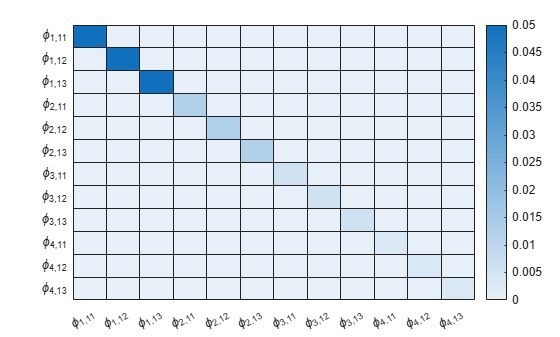For conjugate Bayesian VAR models, scaled covariances are proportional among equations.

Consider the 3-D VAR(4) model of Create Matrix-Normal-Inverse-Wishart Conjugate Prior Model. Estimate the posterior distribution, and generate forecasts from the corresponding posterior predictive distribution.

Load the US macroeconomic data set. Compute the inflation rate. Plot all response series.

load Data_USEconModel seriesnames = ["INFL" "UNRATE" "FEDFUNDS"]; DataTimeTable.INFL = 100*[NaN; price2ret(DataTimeTable.CPIAUCSL)]; figure plot(DataTimeTable.Time,DataTimeTable{:,seriesnames}) legend(seriesnames)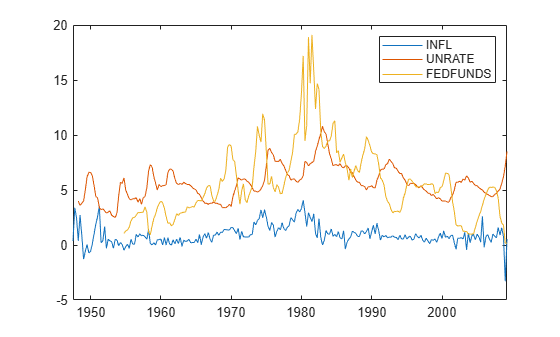Stabilize the unemployment and federal funds rates by applying the first difference to each series.

DataTimeTable.DUNRATE = [NaN; diff(DataTimeTable.UNRATE)]; DataTimeTable.DFEDFUNDS = [NaN; diff(DataTimeTable.FEDFUNDS)]; seriesnames(2:3) = "D" + seriesnames(2:3);

Remove all missing values from the data.

rmDataTimeTable = rmmissing(DataTimeTable);

Create Prior Model

Create a conjugate Bayesian VAR(4) prior model for the three response series. Specify the response variable names.

numseries = numel(seriesnames); numlags = 4; PriorMdl = conjugatebvarm(numseries,numlags,'SeriesNames',seriesnames);

Estimate Posterior Distribution

Estimate the posterior distribution by passing the prior model and entire data series to estimate.

PosteriorMdl = estimate(PriorMdl,rmDataTimeTable{:,seriesnames},'Display','equation');
Bayesian VAR under conjugate priors Effective Sample Size: 197 Number of equations: 3 Number of estimated Parameters: 39 VAR Equations | INFL(-1) DUNRATE(-1) DFEDFUNDS(-1) INFL(-2) DUNRATE(-2) DFEDFUNDS(-2) INFL(-3) DUNRATE(-3) DFEDFUNDS(-3) INFL(-4) DUNRATE(-4) DFEDFUNDS(-4) Constant ------------------------------------------------------------------------------------------------------------------------------------------------------------------------------ INFL | 0.1260 -0.4400 0.1049 0.3176 -0.0545 0.0440 0.4173 0.2421 0.0515 0.0247 -0.1639 0.0080 0.1064 | (0.0713) (0.1395) (0.0366) (0.0810) (0.1490) (0.0386) (0.0802) (0.1467) (0.0400) (0.0838) (0.1385) (0.0369) (0.0774) DUNRATE | -0.0236 0.4440 0.0350 0.0900 0.2295 0.0520 -0.0330 0.0567 0.0010 0.0298 -0.1665 0.0104 -0.0536 | (0.0396) (0.0774) (0.0203) (0.0449) (0.0827) (0.0214) (0.0445) (0.0814) (0.0222) (0.0465) (0.0768) (0.0205) (0.0430) DFEDFUNDS | -0.1514 -1.3408 -0.2762 0.3275 -0.2971 -0.3041 0.2609 -0.6971 0.0130 -0.0692 0.1392 -0.1341 -0.3902 | (0.1517) (0.2967) (0.0777) (0.1722) (0.3168) (0.0820) (0.1705) (0.3120) (0.0851) (0.1782) (0.2944) (0.0785) (0.1646) Innovations Covariance Matrix | INFL DUNRATE DFEDFUNDS ------------------------------------------- INFL | 0.2725 -0.0197 0.1407 | (0.0270) (0.0106) (0.0417) DUNRATE | -0.0197 0.0839 -0.1290 | (0.0106) (0.0083) (0.0242) DFEDFUNDS | 0.1407 -0.1290 1.2322 | (0.0417) (0.0242) (0.1220) 

Because the prior is conjugate for the data likelihood, the posterior is a conjugatebvarm object. By default, estimate uses the first four observations as a presample to initialize the model.

Generate Forecasts from Posterior Predictive Distribution

From the posterior predictive distribution, generate forecasts over a two-year horizon. Because sampling from the posterior predictive distribution requires the entire data set, specify the prior model in forecast instead of the posterior.

fh = 8; FY = forecast(PriorMdl,fh,rmDataTimeTable{:,seriesnames});

FY is an 8-by-3 matrix of forecasts.

Plot the end of the data set and the forecasts.

fp = rmDataTimeTable.Time(end) + calquarters(1:fh); figure plotdata = [rmDataTimeTable{end - 10:end,seriesnames}; FY]; plot([rmDataTimeTable.Time(end - 10:end); fp'],plotdata) hold on plot([fp(1) fp(1)],ylim,'k-.') legend(seriesnames) title('Data and Forecasts') hold off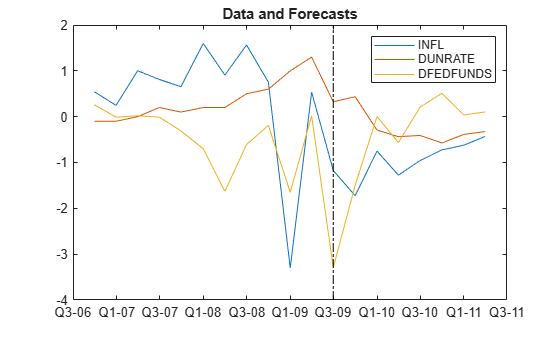Compute Impulse Responses

Plot impulse response functions by passing posterior estimations to armairf.

armairf(PosteriorMdl.AR,[],'InnovCov',PosteriorMdl.Covariance)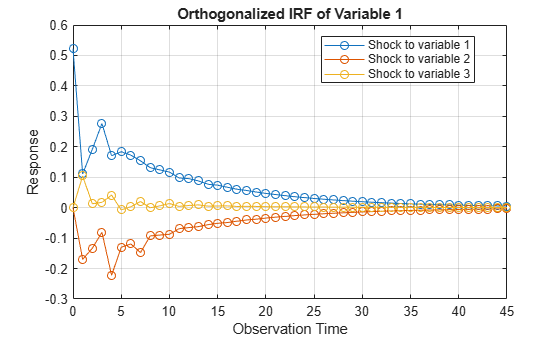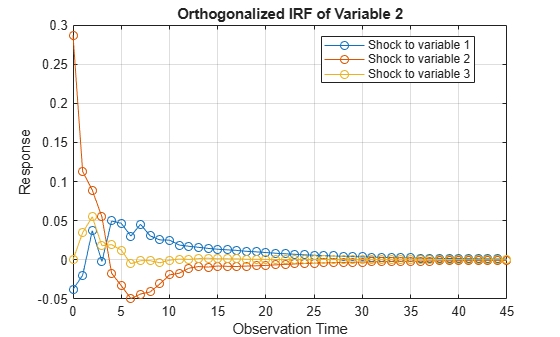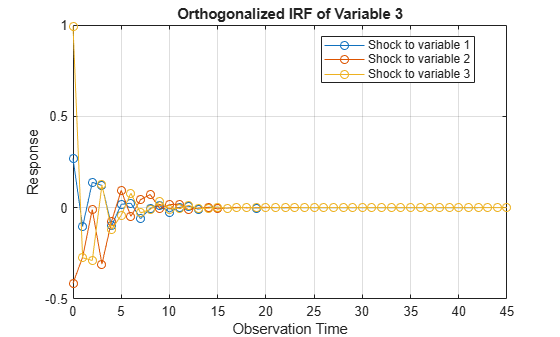expand all

## Algorithms

• If you pass either a conjugatebvarm or diffusebvarm object and data to estimate, MATLAB® returns a conjugatebvarm object representing the posterior distribution.

• The conditional covariance (unscaled) of the entire vectorized matrix normal prior is Σ⊗V. To achieve conjugacy, these conditions must be true:

• Prior covariances are assumed to be proportional among all equations. Σ determines the proportionality, and scales V during posterior estimation.

• For an equation, the covariances between all AR coefficients, self lag and cross lag, are equal.

conjugatebvarm enforces the first condition, but not the second. Therefore, conjugatebvarm applies elements of V to all coefficients in the model relative to the coefficients in the equation of y1,t.

 Litterman, Robert B. "Forecasting with Bayesian Vector Autoregressions: Five Years of Experience." Journal of Business and Economic Statistics 4, no. 1 (January 1986): 25–38. https://doi.org/10.2307/1391384.